# quokar: R package for quantile regression outlier diagnostic

## Abstract

Extensive toolbox for estimation and inference about quantile regression has been developed in the past decades. Recently tools for quantile regresion model diagnostic are studied by researchers. An implementation of outlier diagnostic methods in R language now is available in the package quokar. This vignette offers a brief tutorial introduction to the package. Package quokar is open-source and can be freely downloaded from Github: http://www.github.com/wenjingwang/quokar.

## Introduction

Koenker et al(1978) extended the OLS regression by introducing quantile regression into linear model. In econometrics, social sciences and ecology field, quantile regression methods have been widely used. There are several reasons to use quantile regression: (a) it fully demonstrate the relationship of responsor and predictors on each quantile of the responsor; (b) it relexed the assumptions of classical regression model; (c) estimators of quantile regression have good large sample asymptotic properties.

The distribution function of random variable $$Y$$ can be characterized as

$F(y)=P\{Y \leq y \} \enspace \enspace (1)$

For any $$0<\tau<1$$,

$Q(\tau)=\inf \{y:F(y)\geq \tau\} \enspace \enspace (2)$

is called the $$\tau$$th quantile of $$Y$$. The quantile function provides a complete characterization of $$Y$$.

OLS regression(also called mean regression) is based on the idea of minimizing the euclidean distance between $$y$$ and $$\hat{y}$$. Following this idea, quantile regression trying to minimizing a so called $$\rho_{\tau}$$ distance which is defined as:

$d_{\tau}(y,\hat{y})=\sum_{i=1}^{n}\rho_{\tau}(y_i-\hat{y}_{i})\enspace \enspace (3)$

The linear conditional quantile fucntion, $$Q_{Y}(\tau|\boldsymbol{X}=x)=\boldsymbol{X}^T)\boldsymbol{\beta}_{\tau}$$, can be estimated by solving

$\hat{\boldsymbol{\beta}}_{\tau}=\arg \min_{b \in R^{p}}\sum_{i=1}^{n}{\rho_{\tau}(y_i-x_{i}^{T}\boldsymbol{\beta}_{\tau})} \enspace \enspace (4)$

Extensive toolbox for estimation and inference about quantile regression has been developed. However, few integrate work been done for diagnostics, expecially for outlier diagnostic. We propose outliers of quantile regression are observations that show extreme pattern which can not be explained by the quantile regression model. These observations are leverage points or outliers in y direction, and they may casue bias in the parameter estimates and should be discussed even if its presence is reasonable.

The rest of this vignettes is organized as follows. Section 2 presents how do different locations of outliers affect coefficients of quantile regression on different quantile. Section 3 studied how does quantile regression work and provided data frame for visualizing the results. Section 4 dedicated to introduce the implementation of outlier dignostic methods for quantile regression. Section 5 concludes with a short discussion.

## Outliers affect quantile regression on different quantile

In mean regression practice, we fits one model on the mean of responsor using one dataset. Mean regression can be very sensitive to outliers, which will distort its whole estimation results. In terms of quantile regression, we fits model on each quantile of responsor, and outliers may affect coefficients of fitted model on each quantile corresponds to their location.

In two simple simulation studies, we generate 100 sample observation and 3 outliers. The outliers are distributed in two locations in each case. We fitted mean regression and quantile regression based on these dataset to observe how do the outliers affect model coefficients. The results show that different quantile estimations are affected by outliers differently, and the location of outliers matters.

### Outliers affect regression on high quantile

library(quokar)
library(quantreg)
## Warning: package 'quantreg' was built under R version 3.3.3
## Warning: package 'SparseM' was built under R version 3.3.3
library(ggplot2)
## Warning: package 'ggplot2' was built under R version 3.3.3
library(gridExtra)
## Warning: package 'gridExtra' was built under R version 3.3.3
library(purrr)
## Warning: package 'purrr' was built under R version 3.3.3
library(tidyr)
## Warning: package 'tidyr' was built under R version 3.3.3
library(dplyr)
## Warning: package 'dplyr' was built under R version 3.3.3
library(robustbase)
## Warning: package 'robustbase' was built under R version 3.3.3
x <- sort(runif(100))
y1 <- 40*x + x*rnorm(100, 0, 10)
df <- data.frame(y1, x)
add_outlier <- data.frame(y1 = c(60,61,62), x = c(0.71, 0.73,0.75))
model1 <- lm(y1 ~ x, data = df)
model2 <- lm(y1 ~ x, data = df_o)
coeff_lm <- c(model1$coef, model2$coef)
inter_lm <- c(model1$coef, model2$coef)
flag <- c("without-outlier", "with-outlier")
line_lm <- data.frame(coeff_lm, inter_lm, flag)
ggplot(df_o, aes(x = x, y = y1)) +
geom_point(alpha = 0.1) +
geom_abline(data = line_lm, aes(intercept = inter_lm,
slope = coeff_lm, colour = flag))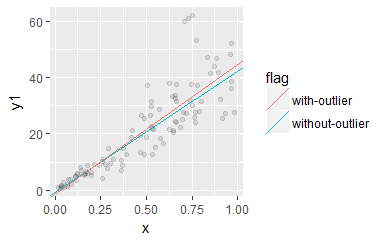Fitting mean regression model using simulated datasets with and without outliers. The outliers are located at the top-left of the original dataset. Results show that outliers pull up the regression line.

coef1 <- rq(y1 ~ x, tau = c(0.1, 0.5, 0.9), data = df, method = "br")$coef rq_coef1 <- data.frame(intercept = coef1[1, ], coef = coef1[2, ], tau_flag =colnames(coef1)) coef2 <- rq(y1 ~ x, tau = c(0.1, 0.5, 0.9),data = df_o, method = "br")$coef
rq_coef2 <- data.frame(intercept = coef2[1, ], coef = coef2[2, ],
tau_flag =colnames(coef2))
ggplot(df_o) +
geom_point(aes(x = x, y = y1), alpha = 0.1) +
geom_abline(data = rq_coef1, aes(intercept = intercept,
slope = coef, colour = tau_flag))+
geom_abline(data = rq_coef2, aes(intercept = intercept,
slope = coef, colour = tau_flag))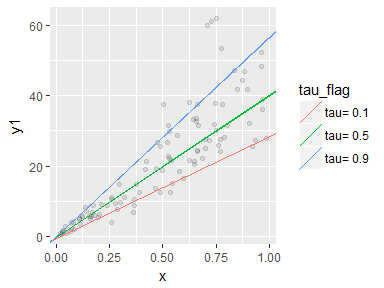Fitting quantile regression model on quantile 0.1, 0.5 and 0.9 using simulated datasets with and without outliers. The outliers are located at the top-left of the original dataset. Results show that outliers pull up the slope of the 0.9 and 0.1 regression line.

### Outliers affect regression on low quantile

x <- sort(runif(100))
y2 <- 40*x + x*rnorm(100, 0, 10)
df <- data.frame(y2, x)
add_outlier <- data.frame(y2 = c(1,2,3), x = c(0.71, 0.73,0.75))
model1 <- lm(y2 ~ x, data = df)
model2 <- lm(y2 ~ x, data = df_o)
coeff_lm <- c(model1$coef, model2$coef)
inter_lm <- c(model1$coef, model2$coef)
flag <- c("without-outlier", "with-outlier")
line_lm <- data.frame(coeff_lm, inter_lm, flag)
ggplot(df_o, aes(x = x, y = y2)) +
geom_point(alpha = 0.1) +
geom_abline(data = line_lm, aes(intercept = inter_lm,
slope = coeff_lm, colour = flag))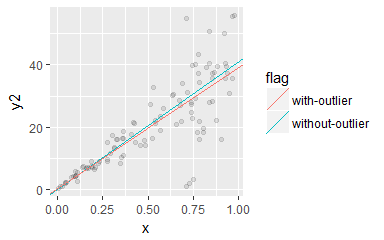Fitting mean regression model using simulated datasets with and without outliers. The outliers are located at the bottom-right of the original dataset. Results show that outliers pull down the slope of the regression line.

coef1 <- rq(y2 ~ x, tau = c(0.1, 0.5, 0.9), data = df, method = "br")$coef rq_coef1 <- data.frame(intercept = coef1[1, ], coef = coef1[2, ], tau_flag = colnames(coef1)) coef2 <- rq(y2 ~ x, tau = c(0.1, 0.5, 0.9), data = df_o, method = "br")$coef
rq_coef2 <- data.frame(intercept = coef2[1, ], coef = coef2[2, ], tau_flag = colnames(coef2))
ggplot(df_o) +
geom_point(aes(x = x, y = y2), alpha = 0.1) +
geom_abline(data = rq_coef1, aes(intercept = intercept,
slope = coef, colour = tau_flag))+
geom_abline(data = rq_coef2, aes(intercept = intercept,
slope = coef, colour = tau_flag))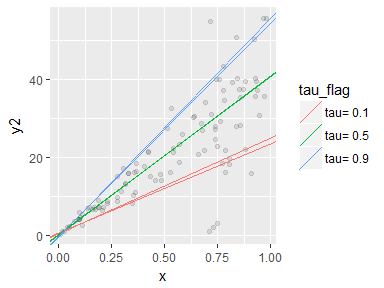Fitting quantile regression model on quantile 0.1, 0.5 and 0.9 using simulated datasets with and without outliers. The outliers are located at the bottom-right of the original dataset. Results show that outliers pull down the slope of the 0.1 regression line.

We also do simulation one step further with different pattern of outliers. First, we generate original dataset, and condaminate the data with outliers. Second, we change the location of outliers or add outliers numbers to observe how they affect coeficient estimations on each quantile. These simulation studies are extended to multi-variables model.

### Outliers moving in y direction

Using the simulated data to construct quantile regression model. By comparing the four models, we have a brief idea of the effect of outliers locating. The results show that when outliers moving down in y direction for 10 unit, it pulls down the slope on every quantile(comparing the result of model rq(y1~x) and rq(y2~x)). However, keeping moving down the outliers does no change to the slopes.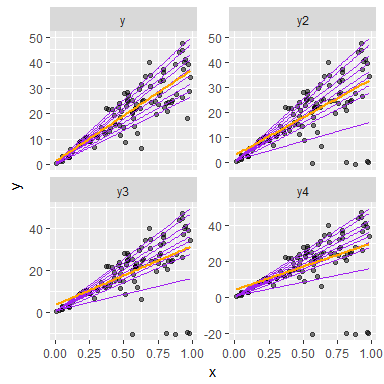Fitting quantile regression models using simulated data. We keep moving down the outliers in y direction in y2 (y-5), y3 (y-10) and y4 (y-15).

To observe how the outliers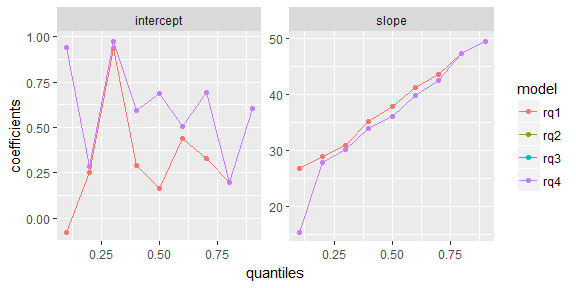Fitting quantile regression models using simulated data. We keep moving down the outliers in y direction getting datasets with variable y2 (=y-5), y3 (=y-10) and y4 (=y-15). Results show that in single predictor case, outliers moving down in y make no difference to the quantile regression coefficients estimations

We also observed the change of coefficients in multi-variable model. The results show that coefficients changes slowly when keep moving down the outliers in y-direction.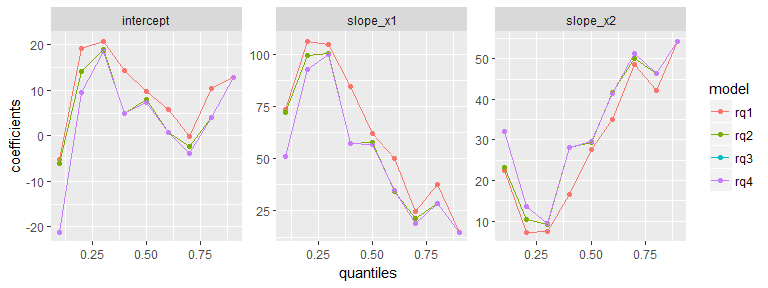Fitting quantile regression models using simulated data. We keep moving down the outliers in y direction getting three datasets with different locations of outliers (changing in y-aixs, y2 (=y-5), y3 (=y-10) and y4 (=y-15)). Results show that in multi predictors case, outliers moving down in y make small change to the quantile regression coefficients estimations

• Outlier move in x direction

If moving outliers in same pattern moving on x direction, slopes change every time outlier moves. To go further, each move does different effect on different quantiles.

x <- sort(runif(100))
y <- 40*x + x*rnorm(100, 0, 10)
selectedIdx <- sample(50:100,5)
df <- data.frame(y)
df$y2 <- y df$x <- x
df$y2[selectedIdx] <- df$x[1:5]*rnorm(5, 0, 10)
df$x2 <- x df$x2[selectedIdx] <- df$x2[selectedIdx] + 0.2 df$x3 <- df$x2 df$x3[selectedIdx] <- df$x3[selectedIdx] + 0.2 df$x4 <- df$x3 df$x4[selectedIdx] <- df$x4[selectedIdx] + 0.2 df_m <- df %>% gather(variable, value, -y, -y2) ggplot(df_m, aes(x = value, y=y2)) + geom_point() + xlab("x") + ylab("y") + facet_wrap(~variable, ncol=2, scale = "free") + geom_quantile(quantiles = seq(0.1, 0.9, 0.1))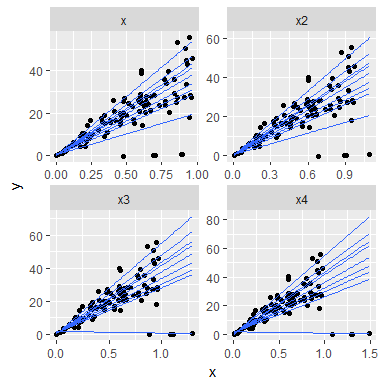Fitting quantile regression models using simulated data. We keep moving the outliers to the right in x direction getting three datasets with different locations of outliers (changing in x-aixs, x2 (=x+0.2), x3 (=x+0.4) and x4 (=x+0.6)). coefs <- 3:6 %>% map(~ rq(df$y2 ~ df[, .], data = df, seq(0.1, 0.9, 0.1))) %>%
map_df(~ as.data.frame(t(as.matrix(coef(.)))))
colnames(coefs) <- c("intercept", "slope")
tau <- rep(seq(0.1, 0.9, by = 0.1), 4)
model <- paste('rq', rep(1:4, each = 9), sep="")
df_m1 <- data.frame(model, tau, coefs)
df_mf <- df_m1 %>% gather(variable, value, -c(model, tau))
ggplot(df_mf, aes(x = tau, y = value, colour = model)) +
geom_point() +
geom_line() +
facet_wrap(~ variable, scale = "free_y") +
xlab('quantiles') +
ylab('coefficients')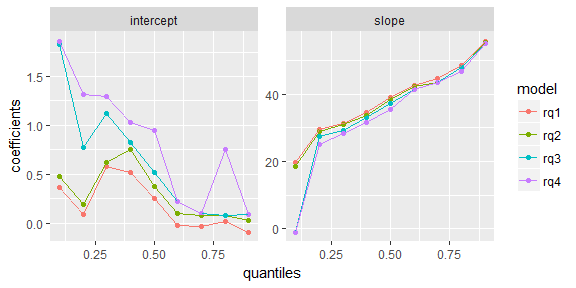Fitting quantile regression models using simulated data. We keep moving the outliers to the right in x direction getting three datasets with different locations of outliers (changing in x-aixs, x2 (=x+0.2), x3 (=x+0.4) and x4 (=x+0.6)).Results show that in single predictors case, outliers moving right in x make significant change to the quantile regression coefficients estimations.

As to the ‘exact fit’ character of estimation process of quantile regression, the number of outliers should be an important factor influencing the robustness of the model. Our simulation result proved this assumption. With growing numbers of outliers, the slopes on each quantile changes.

x <- sort(runif(100))
y <- 40*x + x*rnorm(100, 0, 10)
selectedX1 <- sample(50:100, 5)
y_number_y1 <- y
y_number_y1[selectedX1] <- x[1:5]*rnorm(5, 0, 10)
selectedX2 <- sample(50:100, 10)
y_number_y2 <- y
y_number_y2[selectedX2] <- x[1:10]*rnorm(10, 0, 10)
selectedX3 <- sample(50:100, 15)
y_number_y3 <- y
y_number_y3[selectedX3] <- x[1:15]*rnorm(15, 0, 10)
df <- data.frame(x, y, y_number_y1, y_number_y2, y_number_y3)
df_m <- df %>% gather(variable, value, -x)
ggplot(df_m, aes(x=x, y=value)) +
geom_point() +
xlab("x") +
ylab("y") +
facet_wrap(~variable, ncol=2) +
geom_quantile(quantiles = seq(0.1, 0.9, 0.1))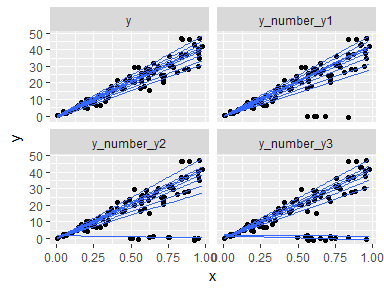Fitting quantile regression models using simulated data. We keep add numbers of outliers to the original data getting three datasets with different numbers of outliers (number of outliers are 5, 10 and 15). Results show that in single predictors case, more numbers of outliers pulled more quantile regression lines down.

• Real data example

We also explored the Australia Sports Institute data to observe if it contains outliers in fitting quantile regression models. This dataset contain 100 observations and 13 variables. We use this data to explore how do LBM, Bfat and Ferr affect the BMI of human body. Based on scatter plot of the responsor and predictor, we got suspicious outlier point 75. And the following results show the changing of fitting lines when leaving this point out.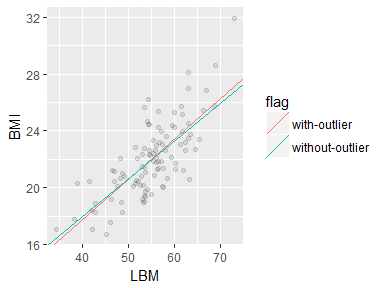Fitting mean regression using ais data with and without case 75, which is a suspicious outlier. Results show that the slope of mean regression line will be smaller when leaveing case 75 out.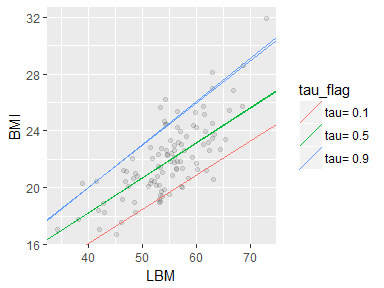Fitting quantile regression using ais data with and without case 75, which is a suspicious outlier. Results show that the slope of 0.9 and 0.5 quantile regression line will be smaller when leaveing case 75 out.

## How does quantile regression work: observations used in quantile regression fitting

Basically, quantile regression has no distribution assummptions on its error term. Computation of quantile regression estimators may be formulated as a linear programming problem and efficiently solved by simplex or barrier methods. The former is used for relative small sample size, and the latter can deal with large sample size data (n>1000).

Both methods can be conviniently applied to get quantile regression estimators using package quantreg. While unfortunately, related functions do not provide detailed results on how do these algorithms working, and different observations used when fitting different quantiles remained in the blackbox. In this section, we introduce functions in quokar aimed to unfold the blackbox of the fitting process of quantile regression, and visualize the results to offer a much easier way to understand those algorithms.

### Simplex Method: exact-fit

The simplex method for quantile regression fitting is closely related to linear programming and typically yields a solution on the vertax of the solution polygon. The ‘exact-fit’ property of simplex method may confuse people for considering the reliability of quantile regression model for its ignoring so many observations in the dataset. While those data not used in getting the final estimation result are involved in locating the optimum vertax.

Function frame-br returns the indice of sample observations used in quantile regression fitting.

### Interior Point Method: 0-1 weighting

Interior point method extend simplex method for dealing large sample size data. Stephen $$\&$$ Roger(1997) introduced this algrithm to quantile regression model to do coefficient inference. This algorithm put 0-1 weights on the observations, which in nature is the same with simplex method.

Function frame_fn_obs returns the observations weighted 1 in interior point method.

tau <- c(0.1, 0.5, 0.9)
fn <- rq(BMI ~ LBM, data = ais_female, tau = tau, method = 'fn')
fn_obs <- frame_fn_obs(fn, tau)
head(fn_obs)
##            tau0.1       tau0.5       tau0.9
## [1,] 3.463266e-10 8.032508e-11 1.294102e-09
## [2,] 3.676714e-08 1.552504e-10 2.581219e-09
## [3,] 3.680850e-11 1.659972e-08 9.871077e-09
## [4,] 5.848082e-11 1.532711e-09 6.737070e-09
## [5,] 5.315053e-09 9.057593e-11 2.478669e-09
## [6,] 5.902536e-11 1.364510e-09 7.639299e-09
fn1 <- fn_obs[,1]
case <- 1: length(fn1)
fn1 <- cbind(case, fn1)
m <- data.frame(y = ais_female$BMI, x1 = ais_female$LBM,fn1)
p <- length(attr(fn$coefficients, "dimnames")[]) m_f <- m %>% gather(variable, value, -case, -fn1, -y) mf_a <- m_f %>% group_by(variable) %>% arrange(variable, desc(fn1)) %>% filter(row_number() %in% 1:p) p1 <- ggplot(m_f, aes(x = value, y = y)) + geom_point(alpha = 0.1) + geom_point(data = mf_a, size = 3, colour = "purple") + facet_wrap(~variable, scale = "free_x") + xlab("x") fn2 <- fn_obs[,2] case <- 1: length(fn2) fn2 <- cbind(case, fn2) m <- data.frame(y = ais_female$BMI, x1 = ais_female$LBM, fn2) p <- length(attr(fn$coefficients, "dimnames")[])
m_f <- m %>% gather(variable, value, -case, -fn2, -y)
mf_a <- m_f %>%
group_by(variable) %>%
arrange(variable, desc(fn2)) %>%
filter(row_number() %in% 1:p )
p2 <- ggplot(m_f, aes(x = value, y = y)) +
geom_point(alpha = 0.1) +
geom_point(data = mf_a, size = 3, colour = "blue", alpha = 0.5) +
facet_wrap(~variable, scale = "free_x") +
xlab("x")
fn3 <- fn_obs[ ,3]
case <- 1: length(fn3)
fn3 <- cbind(case, fn3)
m <- data.frame(y = ais_female$BMI, x1 = ais_female$LBM,
fn3)
p <- length(attr(fn$coefficients, "dimnames")[]) m_f <- m %>% gather(variable, value, -case, -fn3, -y) mf_a <- m_f %>% group_by(variable) %>% arrange(variable, desc(fn3)) %>% filter(row_number() %in% 1:p ) p3 <- ggplot(m_f, aes(x = value, y = y)) + geom_point(alpha = 0.1) + geom_point(data = mf_a, size = 3, colour = "orange") + facet_wrap(~variable, scale = "free_x") + xlab("x") grid.arrange(p1, p2, p3, ncol = 3)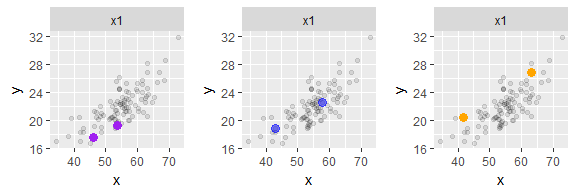This plot shows the weighting scheme in quantile regression using interior point method. On each quantile, the sum weights of coloured points is 1, and other points are weighted 0. ## --- fn-weights1 tau <- c(0.1, 0.5, 0.9) fn <- rq(BMI ~ LBM, data = ais_female, tau = tau, method = 'fn') fn_obs <- frame_fn_obs(fn, tau) p <- 2 obs <- data.frame(cbind(fn_obs,id = 1:nrow(fn_obs))) selected <- NULL for(i in 1:3){ data <- obs[order(obs[,i],decreasing = T),c(i,4)][1:p,] data <- cbind(data,idx=1:p) colnames(data) <- c("value","id","idx") data = cbind(data,type=rep(colnames(obs)[i],p)) if(is.null(selected)){ selected = data }else{ selected = rbind(selected,data) } } selected$value = round(selected$value,3) ggplot(selected,aes(x=idx,y=value,colour=type))+ geom_point(aes(size=value),alpha=0.5)+ geom_text(aes(label = id), hjust = 0, vjust= 0)+ facet_wrap( ~ type,scale="free_y")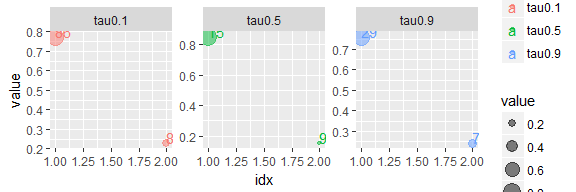This plot shows the weighting scheme in quantile regression using interior point method. On each quantile, the sum weights of coloured points is 1, and other points are weighted 0. ### Non-linear case: smooth weighting Koenker(1996) provided an algorithm for computing quantile regression estimates for problems in which the response function is non-linear in parameters. The basic idea is weigthing, while in a smoother way comparing to linear case. We display the weighting result in R using function nlrq. x <- rep(1:25, 20) y <- SSlogis(x, 10, 12, 2) * rnorm(500, 1, 0.1) Dat <- data.frame(x = x, y = y) formula <- y ~ SSlogis(x, Aysm, mid, scal) nlrq_m <- frame_nlrq(formula, data = Dat, tau = c(0.1, 0.5, 0.9)) weights <- nlrq_m$weights
m <- data.frame(Dat, weights)
m_f <- m %>% gather(tau_flag, value, -x, -y)
ggplot(m_f, aes(x = x, y = y, colour = tau_flag)) +
geom_point(aes(size = value), alpha = 0.5) +
facet_wrap(~tau_flag)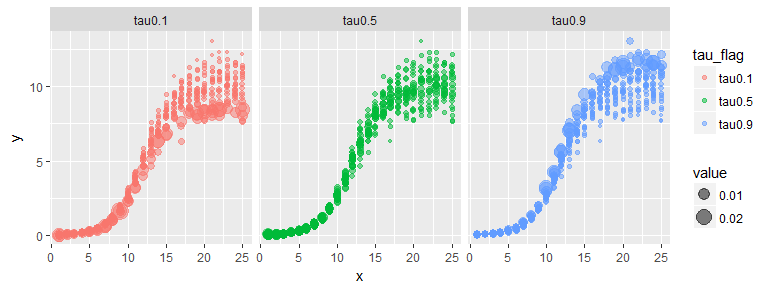Weights put on observations in Non-linear Quantile Regression

### Quantile regression using asymmetric Laplace distribution

Komunjej(2005) noted that likelihood should belong a tick-exponential family. Some researchers use asymmetric Laplace distribution as the assumption distribution of quatnile regression model, which produced different ways of estimating model coefficients. The estimation method

Likelihood of quantile regression model using asymmetric Laplace distribution with pdf as follows:

$f_{ALD}(u)=\frac{\tau(1-\tau)}{\sigma}exp(-\rho_{\tau}(\frac{y-u}{\sigma}))$

where $$\tau$$, $$\sigma$$ and $$\mu$$ are the skew, scale and location parameters.

ALD$$(\mu, \sigma, \tau)$$ is skewed to left when $$\tau>0.5$$, and skewed to right when $$\tau<0.5$$.

Function frame_ald returns the ALD distributions used in quantile regression fit on separate quantiles.

x <- matrix(ais_female$LBM, ncol = 1) y <- ais_female$BMI
tau = c(0.1, 0.5, 0.9)
ald_data <- frame_ald(y, x, tau, smooth = 10, error = 1e-6,
iter = 2000)
ggplot(ald_data) +
geom_line(aes(x = r, y = d, group = obs, colour = tau_flag)) +
facet_wrap(~tau_flag, ncol = 1,scales = "free_y") +
xlab('') +
ylab('Asymmetric Laplace Distribution Density Function')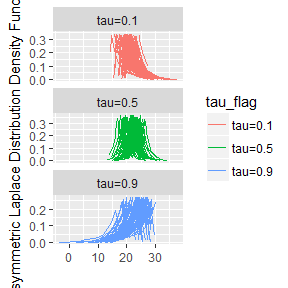Asymmetric Laplace distribution of quantile regression on quantile 0.1, 0.5 and 0.9

Bayesian quantile regression is also based on asymmetric Laplace distribution, and it provide another quantile regression estimating method.

## Outlier Dignostic Methods for Quantile Regression Model

### Residual-Robust Distance Plot

Robust distance is defined as:

$RD(x_i)=[(x_{i}-T(A))^{T}C(A)^{-1}(x_{i}-T(A))]^{1/2}$

where $$T(A)$$ and $$C(A)$$ are robust multivariate location and scale estimates computed with the minimum covariance determinant(MCD) method of Rousseeuw and Van Driessen(1999).

Residuals $$r_i, i = 1,...,n$$ based on quantile regression estimates are used to detect vertical outliers. Outliers are defined as:

OUTLIER=\left\{\begin{aligned}&0 \quad &if \ |r_i| \leq k\sigma \\&1 \quad &otherwise\end{aligned}\right.

where $$\sigma$$ is computed as the corrected median of the absolute residuals $$\sigma=\text{median}\{|r_i|/\phi^{-1}(0.95), i = 1,...,n\}$$

ais_female <- subset(ais, Sex == 1)
tau <- c(0.1, 0.5, 0.9)
object <- rq(BMI ~ LBM + Bfat, data = ais_female, tau = tau)
plot_distance <- frame_distance(object, tau = c(0.1, 0.5, 0.9))
distance <- plot_distance[]
head(distance)
##          md        rd tau_flag  residuals
## 1 1.2275233 1.3912428   tau0.1 -1.4630550
## 2 0.6988854 0.6486756   tau0.1 -0.9262022
## 3 0.3836449 0.3315911   tau0.1  1.0706377
## 4 1.0715694 0.9140188   tau0.1  0.0000000
## 5 0.2538178 0.3814667   tau0.1 -1.0116949
## 6 0.4161890 0.4065418   tau0.1  1.4151942
cutoff_v <- plot_distance[]
cutoff_v
##  2.716203
cutoff_h <- plot_distance[]
cutoff_h
##  12.450378  6.917875 14.073312
n <- nrow(object$model) case <- rep(1:n, length(tau)) distance <- cbind(case, distance) distance$residuals <- abs(distance$residuals) distance1 <- distance %>% filter(tau_flag == 'tau0.1') p1 <- ggplot(distance1, aes(x = rd, y = residuals)) + geom_point() + geom_hline(yintercept = cutoff_h, colour = "red") + geom_vline(xintercept = cutoff_v, colour = "red") + geom_text(data = subset(distance1, residuals > cutoff_h| rd > cutoff_v), aes(label = case), hjust = 0, vjust = 0) + xlab("Robust Distance") + ylab("|Residuals|") distance2 <- distance %>% filter(tau_flag == 'tau0.5') p2 <- ggplot(distance1, aes(x = rd, y = residuals)) + geom_point() + geom_hline(yintercept = cutoff_h, colour = "red") + geom_vline(xintercept = cutoff_v, colour = "red") + geom_text(data = subset(distance1, residuals > cutoff_h| rd > cutoff_v), aes(label = case), hjust = 0, vjust = 0) + xlab("Robust Distance") + ylab("|Residuals|") distance3 <- distance %>% filter(tau_flag == 'tau0.9') p3 <- ggplot(distance1, aes(x = rd, y = residuals)) + geom_point() + geom_hline(yintercept = cutoff_h, colour = "red") + geom_vline(xintercept = cutoff_v, colour = "red") + geom_text(data = subset(distance1, residuals > cutoff_h| rd > cutoff_v), aes(label = case), hjust = 0, vjust = 0) + xlab("Robust Distance") + ylab("|Residuals|") grid.arrange(p1, p2, p3, ncol = 3)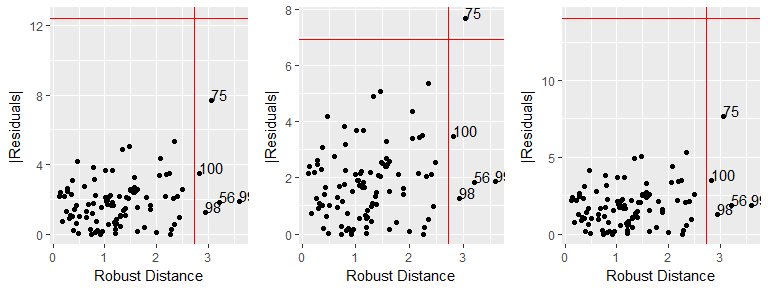Robust Distance-Residual Plot. Points on the right of vertical cutoff line are considered leverage points and points above the horizental cutoff line are outliers in y-direction. ### Generalized Cook Distance To assess the influence of the $$i$$th case on the coefficient estimation of quantile regression, we compare the difference between $$\hat{\theta}_{[i]}$$ and $$\hat{\theta}$$. Case-deletion is a classical approach to study the effects of dropping the $$i$$th observation deleted. Thus, the complete-data log-likelihood function based on the data with the $$i$$th case deleted will be denoted by $$L_{c}(\theta|y_{c[i]})$$. Let $$\hat{\theta}_{[i]}=(\hat{\beta}^{T}_{p[i]}, \hat{\sigma}^{2}_{[i]})^{T}$$ be the maximizer of the function $$Q_{[i]}(\theta|\hat{\theta})=E_{\hat{\theta}}[l_{c}(\theta|Y_{c[i]})|y]$$, where $$\hat{\theta}=(\hat{\beta}^{T}, \hat{\sigma}^{2})^{T}$$ is the ML estimate of $$\theta$$. To calculate the case-deletion estimate $$\hat{\theta}_{[i]}$$ of $$\theta$$, proposed the following one-step approximation based on Q-function, $\hat{\theta}_{[i]}=\hat{\theta}+\{-Q(\hat{\theta}|\hat{\theta})\}^{-1}Q_{[i]}(\hat{\theta}|\hat{\theta})$ where $Q(\hat{\theta}|\hat{\theta})=\frac{\partial^{2}Q(\theta|\hat{\theta})}{\partial\theta\partial \theta^{T}}|_{\theta=\hat{\theta}}$ $Q_{[i]}(\hat{\theta}|\hat{\theta})=\frac{\partial Q_{[i]}(\theta|\hat{\theta})}{\partial\theta}|_{\theta=\hat{\theta}}$ are the Hessian matrix and the gradient vector evaluated at $$\hat{\theta}$$, respectively. For measuring the distance between $$\hat{\theta}_{[i]}$$ and $$\hat{\theta}$$. We consider generalized cook distance as follows. $GD_{i} =(\hat{\theta}_{[i]}-\hat{\theta})^{T}\{-Q(\hat{\theta}|\hat{\theta})\}(\hat{\theta}_{[i]}-\hat{\theta}), i=1,...,n$ ais_female <- subset(ais, Sex == 1) y <- ais_female$BMI
x <- cbind(1, ais_female$LBM, ais_female$Bfat)
tau <- c(0.1, 0.5, 0.9)
case <- rep(1:length(y), length(tau))
GCD <- frame_mle(y, x, tau, error = 1e-06, iter = 10000,
method = 'cook.distance')
GCD_m <- cbind(case, GCD)
ggplot(GCD_m, aes(x = case, y = value )) +
geom_point() +
facet_wrap(~variable, scale = 'free_y') +
geom_text(data = subset(GCD_m, value > mean(value) + 2*sd(value)),
aes(label = case), hjust = 0, vjust = 0) +
xlab("case number") +
ylab("Generalized Cook Distance")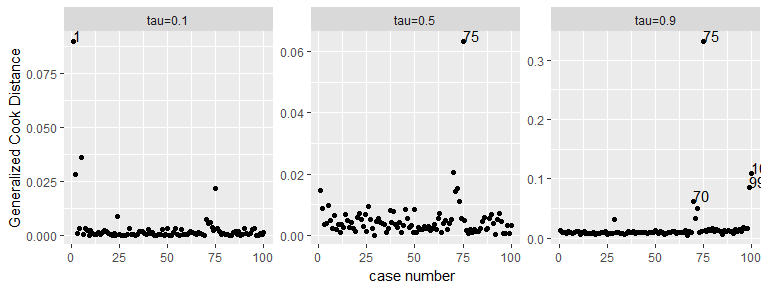Generalized cook distance of each observation on quantile 0.1, 0.5 and 0.9. Case 75 has relative large cook distance funtion distance to other points

### Q Function Distance

The measurement of the influence of the $$i$$th case is based on the Q-distance function, similar to the likelihood distance $$LD_{i}$$, defined as

$QD_{i}=2\{Q(\hat{\theta}|\hat{\theta})-Q(\hat{\theta}_{[i]}|\hat{\theta})\}$

QD <- frame_mle(y, x, tau, error = 1e-06, iter = 100,
method = 'qfunction')
QD_m <- cbind(case, QD)
ggplot(QD_m, aes(x = case, y = value)) +
geom_point() +
facet_wrap(~variable, scale = 'free_y')+
geom_text(data = subset(QD_m, value > mean(value) + sd(value)),
aes(label = case), hjust = 0, vjust = 0) +
xlab('case number') +
ylab('Qfunction Distance')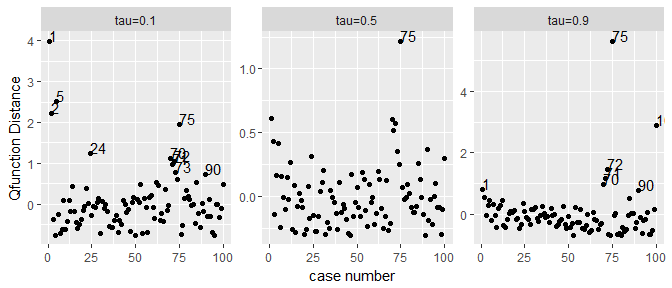Q-function distance of each observation on quantile 0.1, 0.5 and 0.9 from left to right. Case 75 has relative large Q-funtion distance to other points.

### Mean Posterior Probability

Baysian quantile regression added a latent variable $$v_i$$ into model for each observation. Every $$v_i$$ is assumed to have an exponential distribution with mean $$\sigma$$, that with the likelihood produces a posterior distributed according to a generalized inverse Gaussian with parameters.

If we define the variable $$O_i$$, which takes value equal to 1 when the $$i$$th observation is an outlier, and 0 otherwise. Then we propose to calculate the pro bility of an observation being an outlier as

$P(O_i=1)=\frac{1}{n-1}\sum_{j \neq i}P(v_i > v_j|data)$

The probability in the expression above can be approximated given the MCMC draws, as follows:

$P(O_i = 1)=\frac{1}{M}I(v^{(l)_i}>max_{k \in 1:M}v^{(k)}_j)$

where $$M$$ is the size of the chain of $$v_i$$ after the burn-in perior and $$v^{(l)}_i$$ is the $$l$$th draw of this chain.

ais_female <- subset(ais, Sex == 1)
y <- ais_female$BMI x <- matrix(c(ais_female$LBM, ais_female\$Bfat), ncol = 2, byrow = FALSE)
tau <- c(0.1, 0.5, 0.9)
case <- rep(1:length(y), length(tau))
prob <- frame_bayes(y, x, tau, M =  500, burn = 100,
method = 'bayes.prob')
## Current iteration :
##  500
## Current iteration :
##  500
## Current iteration :
##  500
prob_m <- cbind(case, prob)
ggplot(prob_m, aes(x = case, y = value )) +
geom_point() +
facet_wrap(~variable, scale = 'free') +
geom_text(data = subset(prob_m, value > mean(value) + 2*sd(value)),
aes(label = case), hjust = 0, vjust = 0) +
xlab("case number") +
ylab("Mean probability of posterior distribution")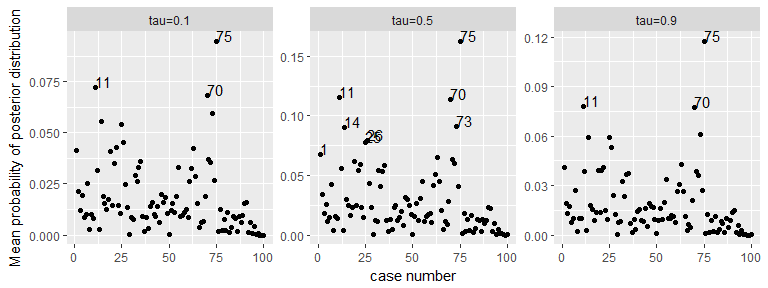Mean posterior probability of each case on quantile 0.1, 0.5 and 0.9. The mean posterior probabilities are calculated based on the postierior distribution of latent variable using Bayesian quantile regression method

### Kullback-Leibler divergence

Similar with mean posterior probability method, we also caculates Kullback-Leibler divergence which proposed by Kullback and Leibler(1951) as a more precise method of measuring the distance between those latent variables in Bayes quantile regression. The Kullback-Leibler divergence is defined as:

$K(f_i, f_j)=\int log(\frac{f_i(x)}{f_j(x)}f_{i}(x))dx$

where $$f_i$$ could be the posterior conditional distribution of $$v_i$$ and $$f_j$$ the posterior conditional distribution of $$v_j$$. We should average this divergence for one observation based on the distance from all others,

$KL(f_i)=\frac{1}{n-1}\sum_{j\neq i}K(f_i, f_j)$

The outliers should show a high probability value for this divergence. We compute the integral using the trapezoidal rule. This method involves MCMC simulation, and it is time consuming. We do not show the result here. You can find the code in file Vignettes.

## Conclusions

Package quokar aimed to provide outlier diagnostic tools for quantile regression using R. It also explored the fitting algorithms used in quantile regression and demonstrated some visualization examples to help understand this blackbox. We also provided useful tools for model visulization using GGobi in our further research. There are more work need to be done for the diagnositc of quantile regression model, expecially for high-dimensional case and extreme high quantiles.